# please solve 2 and 3 1- The reduced stiffness matrix [Q] is given for a unidirectional...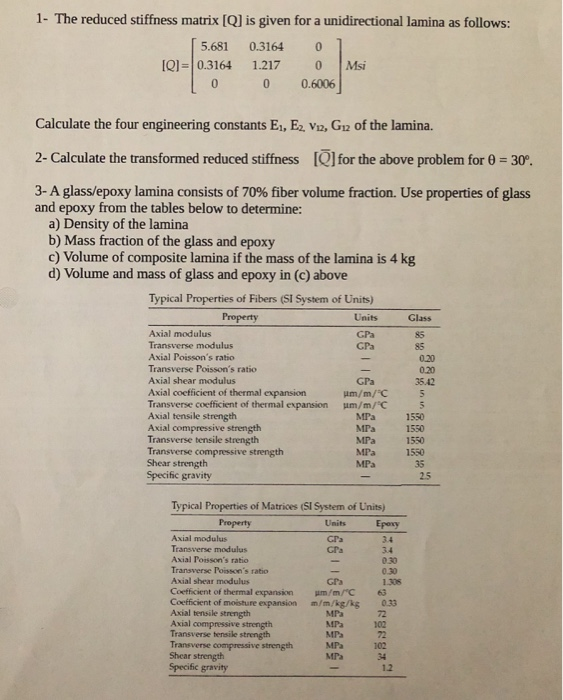1- The reduced stiffness matrix [Q] is given for a unidirectional lamina as follows: 5.681 0.3164 0 ] [Q]=0.3164 1.217 0 Msi I 0 0 0.6006 Calculate the four engineering constants E1, E2, V12, G12 of the lamina. 2- Calculate the transformed reduced stiffness [Q] for the above problem for 0 = 30°. Glass 3- A glass/epoxy lamina consists of 70% fiber volume fraction. Use properties of glass and epoxy from the tables below to determine: a) Density of the lamina b) Mass fraction of the glass and epoxy c) Volume of composite lamina if the mass of the lamina is 4 kg d) Volume and mass of glass and epoxy in (c) above Typical Properties of Fibers (SI System of Units) Property Units Axial modulus Transverse modulus Axial Poisson's ratio 0.20 Transverse Poisson's ratio 0.20 Axial shear modulus GPa 35.42 Axial coefficient of thermal expansion um/m/C Transverse coefficient of thermal expansion m/m/°C Axial tensile strength MPa 1550 Axial compressive strength MPa 1550 Transverse tensile strength MPa Transverse compressive strength MPa 1550 Shear strength MPa Specific gravity 1550 2.5 030 Typical Properties of Matrices (SI System of Units) Property Units Epoxy Axial modulus Cra Transverse modulus GPS Axial Poisson's ratio Transverse Poisson's ratio Axial shear medulus 1305 Coefficient of thermal expansion umm/ Coefficient of moisture expansion m/m/kg/kg 23 Axial tensile strength MPa Axial compressive strength MP Transverse tensile strength MP Transverse compressive strength MPa Shear strength MPa Specific gravity 1.2 100 102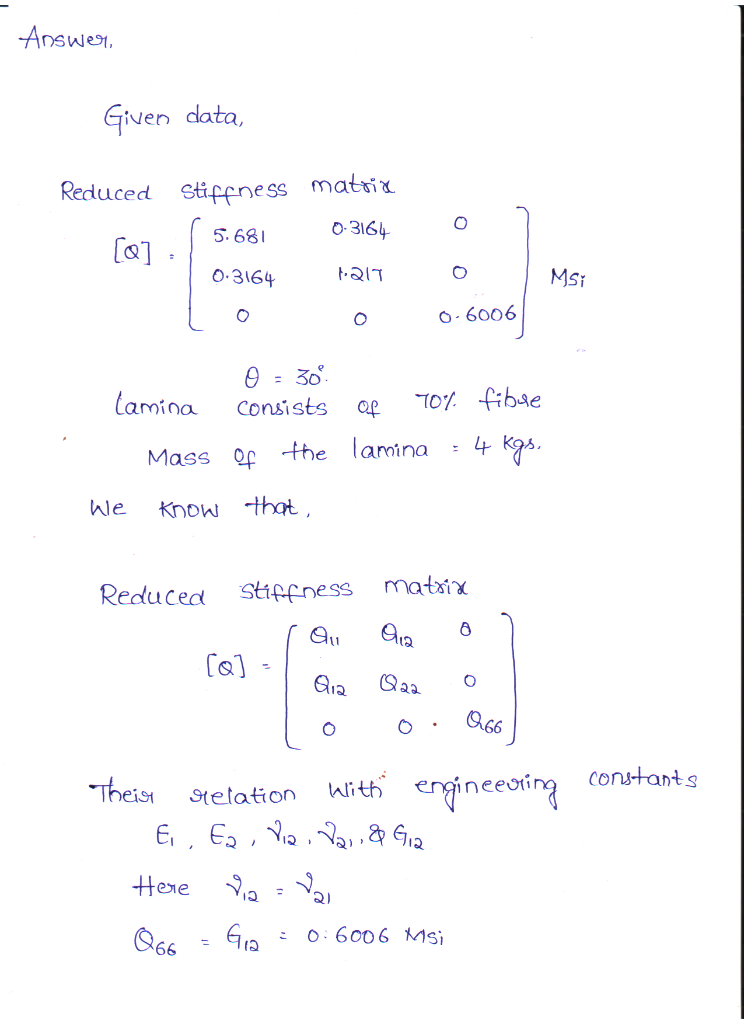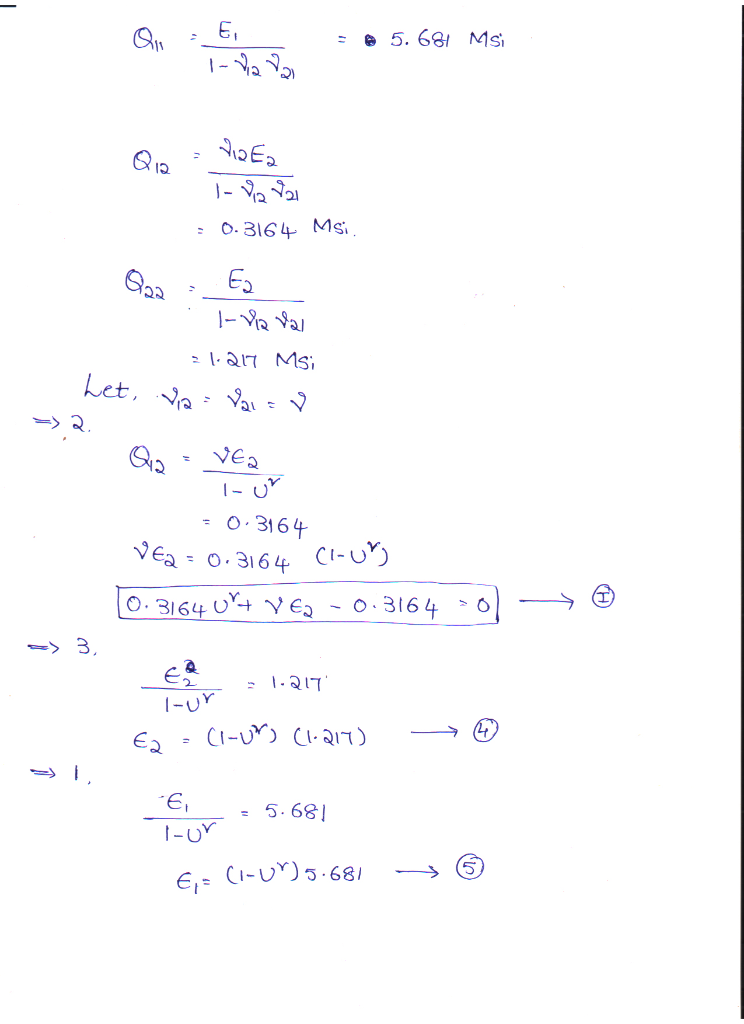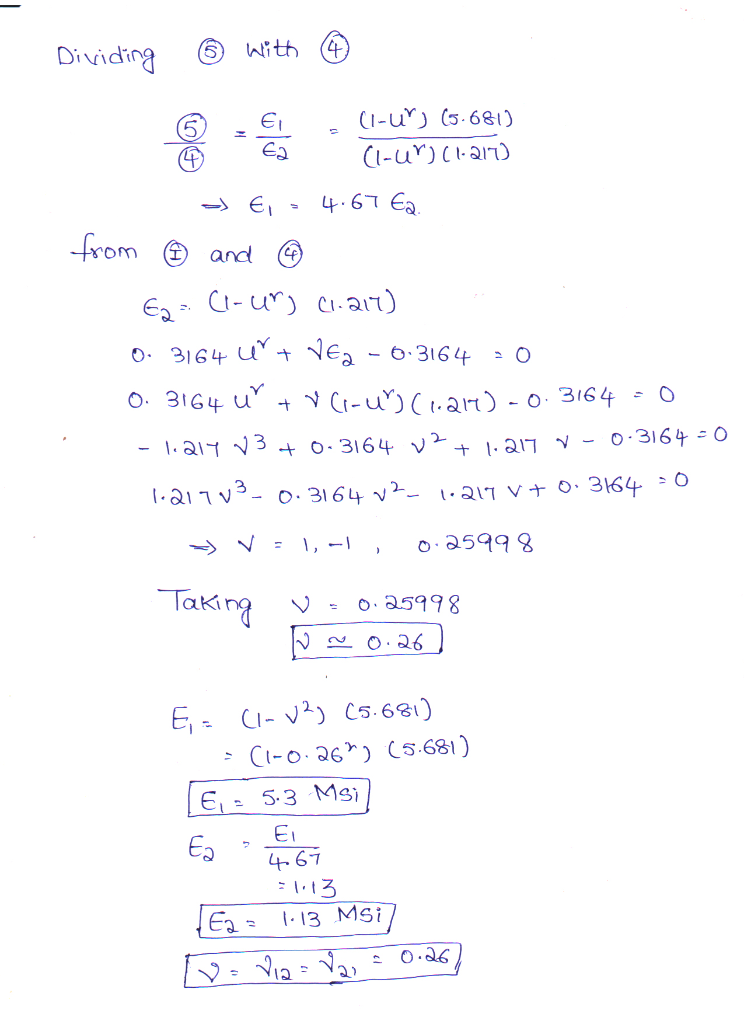##### Add Answer to: please solve 2 and 3 1- The reduced stiffness matrix [Q] is given for a unidirectional...
Similar Homework Help Questions
• ### Composite Structures Tutorial 7 (week 8) 7.1 Find the five strength parameters of a unidirectional glass/epoxy lamina with 40% fiber volume fraction. Use the properties of glass and ep...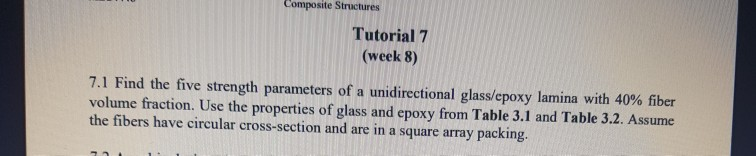Composite Structures Tutorial 7 (week 8) 7.1 Find the five strength parameters of a unidirectional glass/epoxy lamina with 40% fiber volume fraction. Use the properties of glass and epoxy from Table 3.1 and Table 3.2. Assume the fibers have circular cross-section and are in a square array packing. 1-52.0-\$01674870 225 47 Find 208 Mechanics of Composite Materials, Second Edi Convert PO or Excel Orl Select PDF I TABLE 3.1 Typical Properties of Fibers (SI System of Units) Units Graphite Glass...

• ### 235 Find the off-axis shear strength and mode of failure of a 60 boron/ epoxy lamina. Use the pro...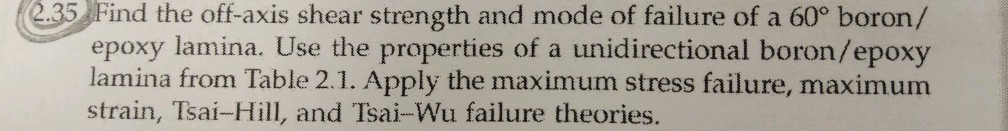235 Find the off-axis shear strength and mode of failure of a 60 boron/ epoxy lamina. Use the properties of a unidirectional boron/epoxy lamina from Table 2.1. Apply the maximum stress failure, maximum strain, Tsai-Hill, and Tsai-Wu failure theories. TABLE 2.1 Tvpical Mechanical Properties of a Unidirectional Lamina (SI System of Units) Glass Boron/ Graphite/ Property SymbolUnits epoxy epoxy epoxy Fiber volume fraction Longitudinal elastic modulus Transverse elastic modulus Major Poisson's ratio Shear modulus Ultimate longitudinal tensile strength Ultimate longitudinal...

• ### 3. A [0/90]s graphite/epoxy laminate is subjected to a tensile normal load of 1N/m in the x- dire...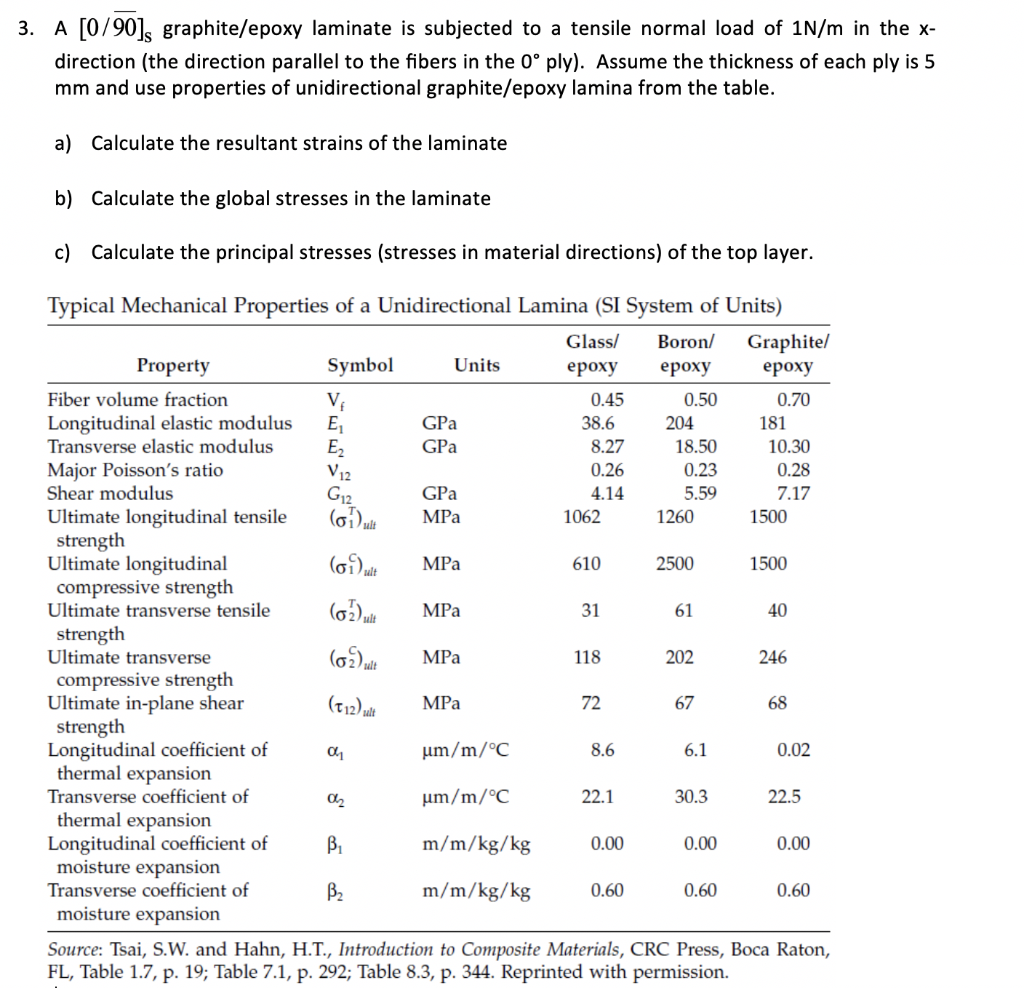3. A [0/90]s graphite/epoxy laminate is subjected to a tensile normal load of 1N/m in the x- direction (the direction parallel to the fibers in the 0° ply). Assume the thickness of each ply is 5 mm and use properties of unidirectional graphite/epoxy lamina from the table. a) Calculate the resultant strains of the laminate b) Calculate the global stresses in the laminate c) Calculate the principal stresses (stresses in material directions) of the top layer. Typical Mechanical Properties of...

• ### 6.1 A simply supported laminated composite beam (Figure 6.6) made of glass/epoxy is 75 mm long an...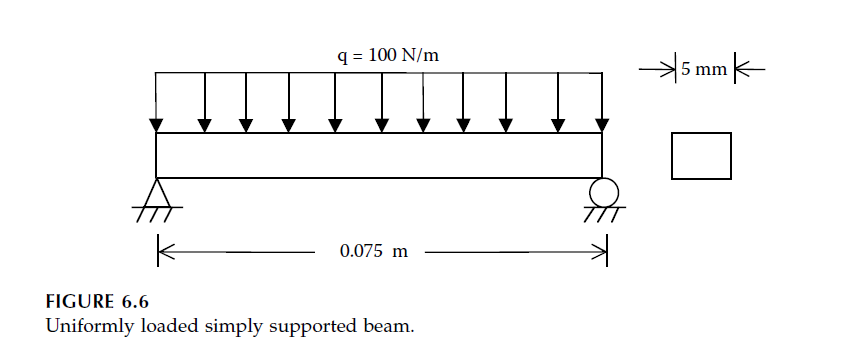6.1 A simply supported laminated composite beam (Figure 6.6) made of glass/epoxy is 75 mm long and has the layup of [±30]2s. A uniform load is applied on the beam that is 5 mm in width. Assume each ply is 0.125 mm thick and the properties of glass/epoxy are from Table 2.1. 1. What is the maximum deflection of the beam? 2. Find the local stresses at the top of the laminate. q- 100 N/m 0.075 m FIGURE 6.6 Uniformly...

• ### 2.15 The reduced stiffness matrix [Q] is given for a unidirectional lamina is given as follows:...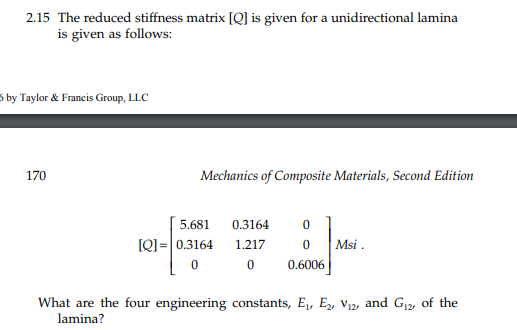2.15 The reduced stiffness matrix [Q] is given for a unidirectional lamina is given as follows: by Taylor & Francis Group, LLC Mechanics of Composite Materials, Second Edition 170 5,681 0.3164 0 Msi Q]= 0.3164 1.217 0 0 0 0.6006 What are the four engineering constants, E E, v12, and G12, of the lamina? 2.15 The reduced stiffness matrix [Q] is given for a unidirectional lamina is given as follows: by Taylor & Francis Group, LLC Mechanics of Composite Materials,...

• ### how to predict how much load can this truss bridge carry? 又 50 0 Comments Value Physical roperties Air Dry...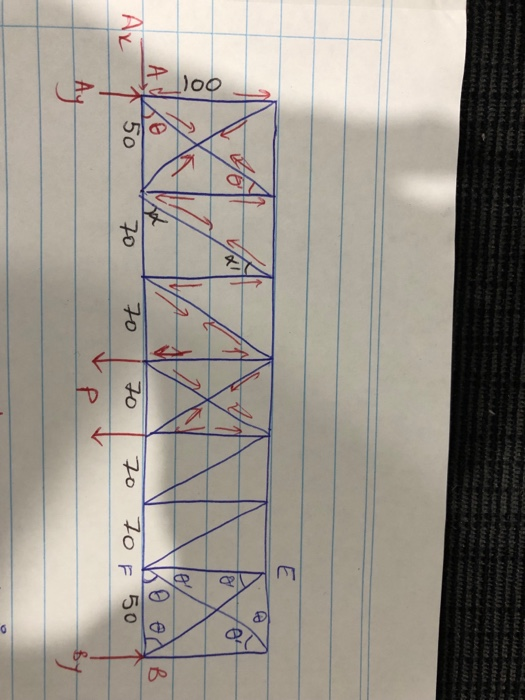how to predict how much load can this truss bridge carry? 又 50 0 Comments Value Physical roperties Air Dry (12-15% Moisture Content) Density 0.160 g/cc Mature wood 2.20 mm Cell Length Comments Value Mechanical Properties Side Hardness, Wood 400 N Indentation Perpendicular to Grain Axial Tensile Strength, 1.00 MPa Ultimate Tensile Strength, 73.0 MPa Ultimate of 0.0190 GPa Static Bending Modulus Rupture Flexural Modulus |2.55 - 3.17 Static Bending GPa Compressive Yield Strength 1.00 MPa Perpendicular to Grain Compressive...

• ### 1. A tensile force of 100 kN is applied on a 0.02 m diameter 2 m...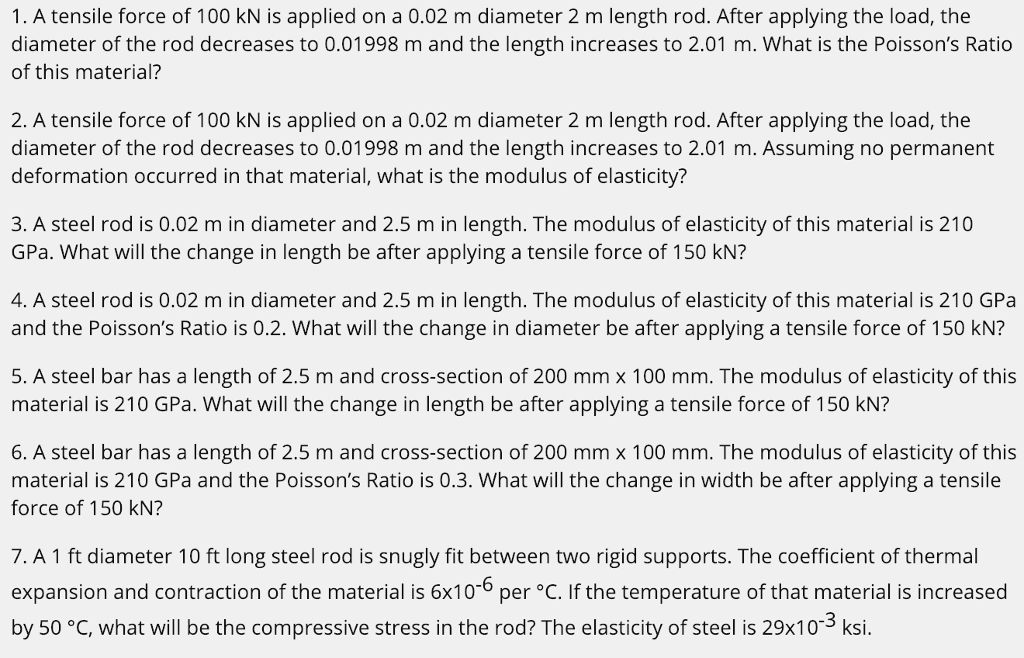1. A tensile force of 100 kN is applied on a 0.02 m diameter 2 m length rod. After applying the load, the diameter of the rod decreases to 0.01998 m and the length increases to 2.01 m. What is the Poisson's Ratio of this material? 2. A tensile force of 100 kN is applied on a 0.02 m diameter 2 m length rod. After applying the load, the diameter of the rod decreases to 0.01998 m and the length...

• ### 6. An alloy rod is used in an application involving wide temperature changes. The alloy has the following properties: (1) elastic modulus of 70 GPa, (2) yield strength of 40 MPa, (3) fracture strengt...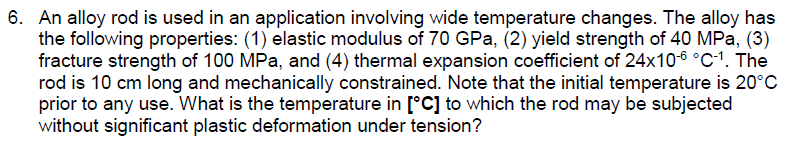6. An alloy rod is used in an application involving wide temperature changes. The alloy has the following properties: (1) elastic modulus of 70 GPa, (2) yield strength of 40 MPa, (3) fracture strength of 100 MPa, and (4) thermal expansion coefficient of 24x10-6 °c-1. The rod is 10 cm long and mechanically constrained. Note that the initial temperature is 20°C prior to any use. What is the temperature in [°C] to which the rod may be subjected without significant...

• ### Please answer all 7 parts lExaminatio The following information applies to questions 1 to 7 -below....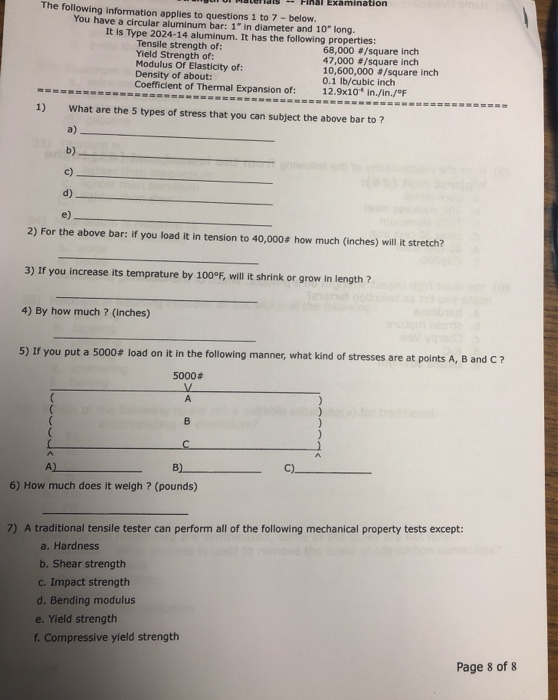Please answer all 7 parts lExaminatio The following information applies to questions 1 to 7 -below. You have a circular aluminum bar: 1 in diameter and 10" long. It is Type 2024-14 aluminum. It has the following properties: Tensile strength of: Yield Strength of: Modulus Of Elasticity of: Density of about: Coefficient of Thermal Expansion of: 12.9x10* in./in./ 68,000 #/square inch 47,000 #/square inch 10,600,000 #/square inch 0.1 lb/cubic inch 1) What are the 5 types of stress that you...

• ### Please Answer this in full detailed as I am unable to get the full grasp of this topic a) Calculate the modulus of a cross-ply HS-carbon/epoxy laminate. Longitudinal fibre modulus (En)-230 GPa; trans...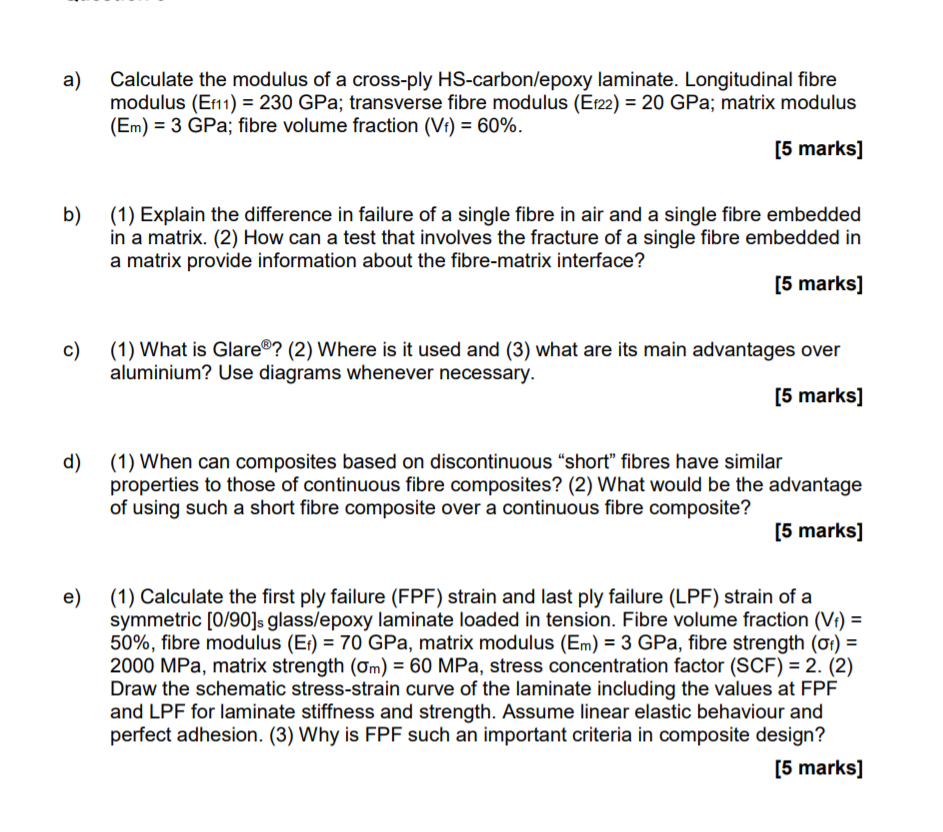Please Answer this in full detailed as I am unable to get the full grasp of this topic a) Calculate the modulus of a cross-ply HS-carbon/epoxy laminate. Longitudinal fibre modulus (En)-230 GPa; transverse fibre modulus (E122)-20 GPa; matrix modulus (En)-3 GPa; fibre volume fraction (V) : 60%. [5 marks] b)(1) Explain the difference in failure of a single fibre in air and a single fibre embedded [5 marks] in a matrix. (2) How can a test that involves the fracture...

Free Homework App